## Example Questions

### Example Question #12 : How To Find The Solution To A Quadratic Equation

2x + y+ xy+ y = x

If y = 1, what is x?

2

0

3

–1

1

–1

Explanation:

Plug in y = 1. Then solve for x.

2x + yxyy = x

2x + 1 + x + 1 = x

3x + 2 = x

2x = -2

x = -1

### Example Question #15 : How To Find The Solution To A Quadratic Equation

What are the-intercept(s) of the following quadratic function?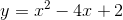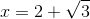and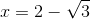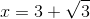and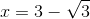and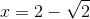and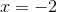andExplanation:-intercepts will occur when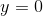. This yields the equation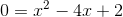We need to use the quadratic formula where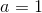,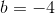and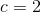.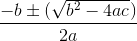Plugging in our values: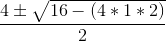Simplifying: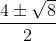Simplifying: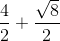Simplifying: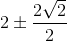Finally: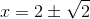### Example Question #19 : Solving Quadratic Equations

The length of a rectangular piece of land is two feet more than three times its width. If the area of the land is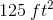, what is the width of that piece of land?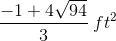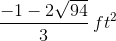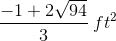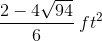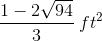Explanation:

The area of a rectangle is the product of its length by its width, which we know to be equal toin our problem. We also know that the length is equal to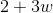, where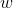represents the width of the land. Therefore, we can write the following equation: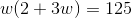Distributing theoutside the parentheses, we get: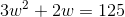Subtractingfrom each side of the equation, we get: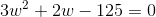We get a quadratic equation, and since there is no factor ofand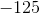that adds up to, we use the quadratic formula to solve this equation.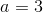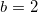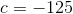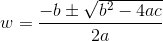We can first calculate the discriminant (i.e. the part under the square root)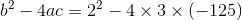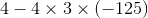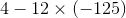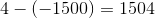We replace that value in the quadratic formula, solving both the positive version of the formula (on the left) and the negative version of the formula (on the right):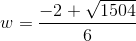Breaking down the square root: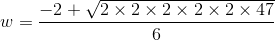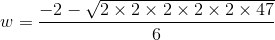We can pull two of the twos out of the square root and place aoutside of it: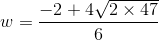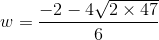We can then multiply theand the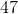: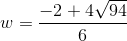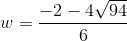At this point, we can reduce the equations, since each of the component parts of their right sides has a factor of: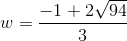Since width is a positive value, the answer is: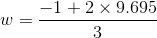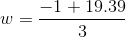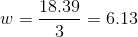The width of the piece of land is approximately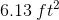.

### Example Question #21 : How To Find The Solution To A Quadratic Equation

Solve for x: x2 + 4x = 5

-1 or 5

-5

-5 or 1

-1

-5 or 1

Explanation:

Solve by factoring.  First get everything into the form Ax2 + Bx + C = 0:

x2 + 4x - 5 = 0

Then factor: (x + 5) (x - 1) = 0

Solve each multiple separately for 0:

X + 5 = 0; x = -5

x - 1 = 0; x = 1

Therefore, x is either -5 or 1

### Example Question #2 : Quadratic Equation

Solve for x: (x2 – x) / (x – 1) = 1

x = -1

x = 2

No solution

x = -2

x = 1

No solution

Explanation:

Begin by multiplying both sides by (x – 1):

x2 – x = x – 1

Solve as a quadratic equation: x2 – 2x + 1 = 0

Factor the left: (x – 1)(x – 1) = 0

Therefore,  x = 1.

However, notice that in the original equation, a value of 1 for x would place a 0 in the denominator.  Therefore, there is no solution.

### Example Question #4 : Quadratic Equation

A farmer has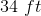of fence, and wants to fence in his sheep. He wants to build a rectangular pen with one side formed by the side of his barn. He wants the area of the pen to be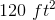. Which of the following is a possible dimension for the side opposite the barn?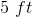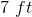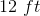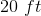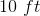Explanation:

Set up two equations from the given information: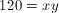and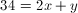.

Substitute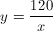into the second equation: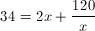Multiply through by.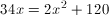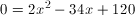Divide by 2: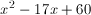We can factor this: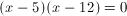Thus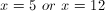.

Note that this is not the side opposite the barn. We need to solve for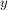in both cases, getting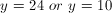.

24 does not appear in the choices, so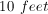is the only possible correct answer.

### Example Question #7 : Quadratic Equation

If f(x) = -x2 + 6x - 5, then which could be the value of a if f(a) = f(1.5)?

3.5
1
2.5
4.5
4
Explanation:

We need to input 1.5 into our function, then we need to input "a" into our function and set these results equal.

f(a) = f(1.5)

f(a) = -(1.5)2 +6(1.5) -5

f(a) = -2.25 + 9 - 5

f(a) = 1.75

-a2 + 6a -5 = 1.75

Multiply both sides by 4, so that we can work with only whole numbers coefficients.

-4a2 + 24a - 20 = 7

Subtract 7 from both sides.

-4a2 + 24a - 27 = 0

Multiply both sides by negative one, just to make more positive coefficients, which are usually easier to work with.

4a2 - 24a + 27 = 0

In order to factor this, we need to mutiply the outer coefficients, which gives us 4(27) = 108. We need to think of two numbers that multiply to give us 108, but add to give us -24. These two numbers are -6 and -18. Now we rewrite the equation as:

4a2 - 6a -18a + 27 = 0

We can now group the first two terms and the last two terms, and then we can factor.

(4a2 - 6a )+(-18a + 27) = 0

2a(2a-3) + -9(2a - 3) = 0

(2a-9)(2a-3) = 0

This means that 2a - 9 =0, or 2a - 3 = 0.

2a - 9 = 0

2a = 9

a = 9/2 = 4.5

2a - 3 = 0

a = 3/2 = 1.5

So a can be either 1.5 or 4.5.

The only answer choice available that could be a is 4.5.

### Example Question #8 : Quadratic Equation

Solve for x:  2(x + 1)2 – 5 = 27

3 or –5

–2 or 5

–2 or 4

3 or 4

–3 or 2

3 or –5

Explanation:

Quadratic equations generally have two answers.  We add 5 to both sides and then divide by 2 to get the quadratic expression on one side of the equation: (x + 1)2 = 16.   By taking the square root of both sides we get x + 1 = –4 or x + 1 = 4.  Then we subtract 1 from both sides to get x = –5 or x = 3.

### Example Question #281 : Equations / Inequalities

Solve 3x2 + 10x = –3

x = –1/6 or –6

x = –2/3 or –2

x = –1/3 or –3

x = –1/9 or –9

x = –4/3 or –1

x = –1/3 or –3

Explanation:

First, the equations must be put in standard form: 3x2 + 10x + 3 = 0

Second, try to factor the quadratic; however, if that is not possible use the quadratic formula.

Third, check the answer by plugging the answers back into the original equation.

### Example Question #1 : How To Find The Solution To A Quadratic Equation

3x2 – 11x = –10

Which of the following is a valid value for x?

3

-5 / 3

5 / 3

-2

5 / 3

Explanation:

Begin by getting our equation into the form Ax2 + BX + C = 0:

3x2 – 11x + 10 = 0

Now, if you factor the left, you can find the answer. Begin by considering the two groups.  They will have to begin respectively with 3 and 1 as coefficients for your x value.  Likewise, looking at the last element, you can tell that both will have to have a + or –, since the C coefficient is positive.  Finally, since the B coefficient is negative, we know that it will have to be –. We know therefore:

(3x – ?)(x – ?)

The potential factors of 10 are: 10, 1; 1, 10; 2, 5; 5, 2

5 and 2 work:

(3x – 5)(x – 2) = 0 because you can FOIL (3x – 5)(x – 2) back into 3x2 – 11x + 10.

Now, the trick remaining is to set each of the factors equal to 0 because if either group is 0, the whole equation will be 0:

3x – 5 = 0 → 3x = 5 → x = 5/3

x – 2 = 0 → x = 2

Therefore, x is either 5 / 3 or 2. The former is presented as an answer.

### All ACT Math Resources